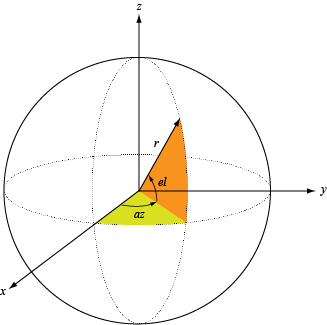Documentation

# patternAzimuth

Plot antenna or transducer element directivity and pattern versus azimuth

## Syntax

``patternAzimuth(element,FREQ)``
``patternAzimuth(element,FREQ,EL)``
``patternAzimuth(element,FREQ,EL,Name,Value)``
``PAT = patternAzimuth(___)``

## Description

````patternAzimuth(element,FREQ)` plots the 2-D element directivity pattern versus azimuth (in dBi) for the element `element` at zero-degrees elevation angle. The argument `FREQ` specifies the operating frequency.```
````patternAzimuth(element,FREQ,EL)`, in addition, plots the 2-D element directivity pattern versus azimuth (in dBi) at the elevation angle specified by `EL`. When `EL` is a vector, multiple overlaid plots are created.```
````patternAzimuth(element,FREQ,EL,Name,Value)` plots the element pattern with additional options specified by one or more `Name,Value` pair arguments.```
````PAT = patternAzimuth(___)` returns the element pattern. `PAT` is a matrix whose entries represent the pattern at corresponding sampling points specified by the `'Azimuth'` parameter and the `EL` input argument.```

## Input Arguments

collapse all

Antenna or transducer element, specified as a Phased Array System Toolbox System object.

Frequency for computing directivity and pattern, specified as a positive scalar. Frequency units are in hertz.

• For an antenna or microphone element, `FREQ` must lie within the range of values specified by the `FrequencyRange` or the `FrequencyVector` property of the element. Otherwise, the element produces no response and the directivity is returned as `–Inf`. Most elements use the `FrequencyRange` property except for `phased.CustomAntennaElement` and `phased.CustomMicrophoneElement`, which use the `FrequencyVector` property.

• For an array of elements, `FREQ` must lie within the frequency range of the elements that make up the array. Otherwise, the array produces no response and the directivity is returned as `–Inf`.

Example: `1e8`

Data Types: `double`

Elevation angles for computing sensor or array directivities and patterns, specified as a 1-by-N real-valued row vector. The quantity N is the number of requested elevation directions. Angle units are in degrees. The elevation angle must lie between –90° and 90°.

The elevation angle is the angle between the direction vector and the xy plane. When measured toward the z-axis, this angle is positive.

Example: `[0,10,20]`

Data Types: `double`

### Name-Value Pair Arguments

Specify optional comma-separated pairs of `Name,Value` arguments. `Name` is the argument name and `Value` is the corresponding value. `Name` must appear inside quotes. You can specify several name and value pair arguments in any order as `Name1,Value1,...,NameN,ValueN`.

Example: `Azimuth,[-90:90],Type,'directivity'`

Displayed pattern type, specified as the comma-separated pair consisting of `'Type'` and one of

• `'directivity'` — directivity pattern measured in dBi.

• `'efield'` — field pattern of the sensor or array. For acoustic sensors, the displayed pattern is for the scalar sound field.

• `'power'` — power pattern of the sensor or array defined as the square of the field pattern.

• `'powerdb'` — power pattern converted to dB.

Example: `'powerdb'`

Data Types: `char`

Azimuth angles, specified as the comma-separated pair consisting of `'Azimuth'` and a 1-by-P real-valued row vector. Azimuth angles define where the array pattern is calculated.

Example: `'Azimuth',[-90:2:90]`

Data Types: `double`

## Output Arguments

collapse all

Element directivity or pattern, returned as an P-by-N real-valued matrix. The dimension P is the number of azimuth values determined by the `'Azimuth'` name-value pair argument. The dimension N is the number of elevation angles, as determined by the `EL` input argument.

collapse all

### Directivity

Directivity describes the directionality of the radiation pattern of a sensor element or array of sensor elements.

Higher directivity is desired when you want to transmit more radiation in a specific direction. Directivity is the ratio of the transmitted radiant intensity in a specified direction to the radiant intensity transmitted by an isotropic radiator with the same total transmitted power

`$D=4\pi \frac{{U}_{\text{rad}}\left(\theta ,\phi \right)}{{P}_{\text{total}}}$`

where Urad(θ,φ) is the radiant intensity of a transmitter in the direction (θ,φ) and Ptotal is the total power transmitted by an isotropic radiator. For a receiving element or array, directivity measures the sensitivity toward radiation arriving from a specific direction. The principle of reciprocity shows that the directivity of an element or array used for reception equals the directivity of the same element or array used for transmission. When converted to decibels, the directivity is denoted as dBi. For information on directivity, read the notes on Element Directivity and Array Directivity.

Computing directivity requires integrating the far-field transmitted radiant intensity over all directions in space to obtain the total transmitted power. There is a difference between how that integration is performed when Antenna Toolbox™ antennas are used in a phased array and when Phased Array System Toolbox antennas are used. When an array contains Antenna Toolbox antennas, the directivity computation is performed using a triangular mesh created from 500 regularly spaced points over a sphere. For Phased Array System Toolbox antennas, the integration uses a uniform rectangular mesh of points spaced 1° apart in azimuth and elevation over a sphere. There may be significant differences in computed directivity, especially for large arrays.

### Azimuth and Elevation Angles

The azimuth angle of a vector is the angle between the x-axis and its orthogonal projection onto the xy-plane. The angle is positive when going from the x-axis toward the y-axis. Azimuth angles lie between –180° and 180° degrees, inclusive. The elevation angle is the angle between the vector and its orthogonal projection onto the xy-plane. The angle is positive when going toward the positive z-axis from the xy-plane. Elevation angles lie between –90° and 90° degrees, inclusive.